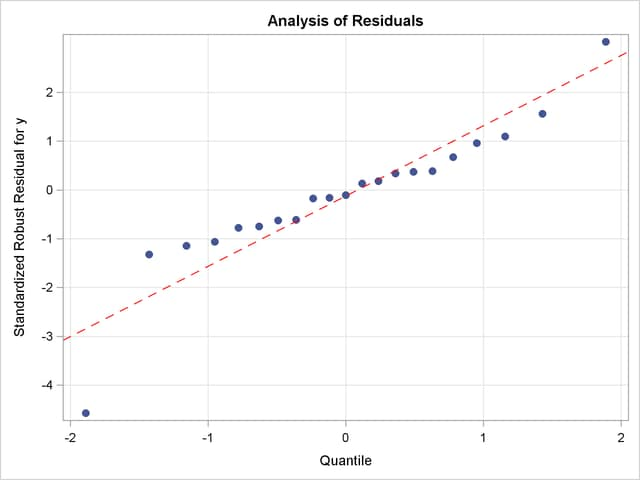Statistical Graphics Using ODS

### Modifying Tick Marks and Grid Lines

This section illustrates how to modify axis tick marks and control grid lines. For example, you can specify the following statement to request tick marks ranging from –4 to 2 in the Y-axis:

```layout Overlay / yaxisopts=(linearopts=(tickvaluelist=(-4 -3 -2 -1 0 1 2)));
```

The LINEAROPTS= option is used for standard linearly scaled axes (as opposed to log-scaled axes). You use the TICKVALUELIST= to specify the tick marks.

You can control the grid lines by using the GRIDDISPLAY= suboption in the YAXISOPTS= option. Typically, you specify either GRIDDISPLAY=AUTO_OFF (grid lines are not displayed unless the GraphGridLines element in the current style contains DisplayOpts="ON") or GRIDDISPLAY=AUTO_ON (grid lines are displayed unless the GraphGridLines element in the current style contains DisplayOpts="OFF"). Here, the template is modified by specifying GRIDDISPLAY=AUTO_ON for both axes. The following statements produce Output 21.5.6:

```proc template;
define statgraph Stat.Robustreg.Graphics.QQPlot;
notes "Q-Q Plot for Standardized Robust Residuals";
dynamic _DEPLABEL Residual;
BeginGraph;
entrytitle "Analysis of Residuals";
layout Overlay / yaxisopts=(gridDisplay=Auto_On
linearopts=(tickvaluelist=(-4 -3 -2 -1 0 1 2))
label=("Standardized Robust Residual for " _DEPLABEL))
xaxisopts=(gridDisplay=Auto_On label="Quantile");
SCATTERPLOT y=eval (SORT(DROPMISSING(RESIDUAL))) x=eval(
PROBIT((NUMERATE(SORT(DROPMISSING(RESIDUAL))) -0.375)/(0.25
+ N(RESIDUAL)))) / primary=true
rolename=(q=eval (
PROBIT((NUMERATE(SORT(DROPMISSING(RESIDUAL))) -0.375)/(0.25
+ N(RESIDUAL)))) s=eval (SORT(DROPMISSING(RESIDUAL))))
tip=(q s) tiplabel=(q="Quantile" s="Residual");
lineparm slope=eval (STDDEV(RESIDUAL)) Y=eval (MEAN(RESIDUAL))
X=0 / lineattrs=GraphReference(color=red pattern=dash)
extend=true;
EndLayout;
EndGraph;
end;
run;

ods graphics on;

proc robustreg data=stack plots=qqplot;
ods select QQPlot;
model y = x1 x2 x3;
run;
```

Output 21.5.6 Q-Q Plot with Modified Y-Axis Tick Marks and GridsYou can restore the default template by running the following step:

```proc template;
delete Stat.Robustreg.Graphics.QQPlot;
run;
```